# 隐马尔可夫模型及其应用（1）简介&解码问题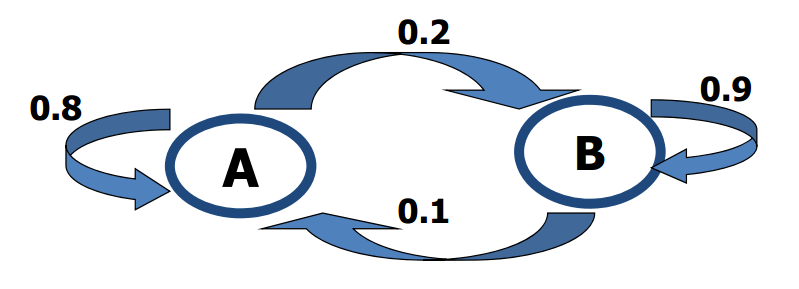HMM有2个状态：

1. 观测状态。我们观察到的骰子序列称为观测状态$\mathbf{Y}=\{y_1,y_2,...,y_T\}$
2. 隐状态。隐含在每个观测状态里面的是隐状态$\mathbf{X}=\{x_1,x_2,...,x_T\}$

T是时间，也可以认为是观测的次数。HMM有3个参数：

1. 初始分布$\mathbf{\mu}=(\mu_i)$$\mu_i=Pr(x_1=i)$，即第一次观测时，每个隐状态出现的概率
2. 转移概率矩阵$A=(a_{ij})$$a_{ij}=Pr(x_{t+1}=j|x_t=i)$，即t时刻的隐状态为i，t+1时刻转移到隐状态j的概率
3. 发射概率矩阵$B=(b_{il})$$b_{il}=Pr(y_t=l|x_t=i)$，即t时候隐状态为i的情况下，观测到状态为l的概率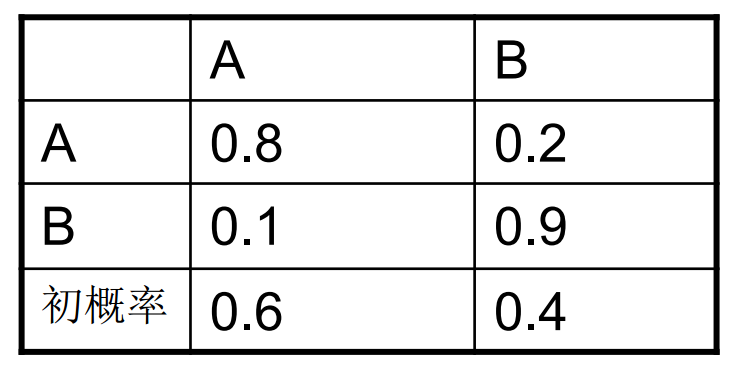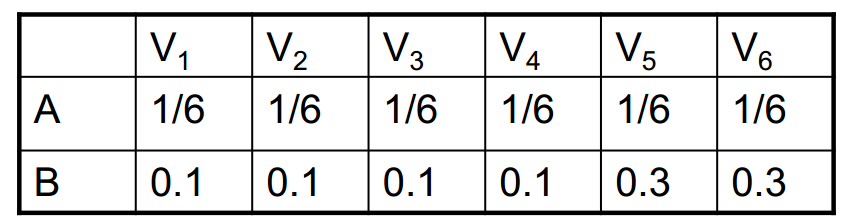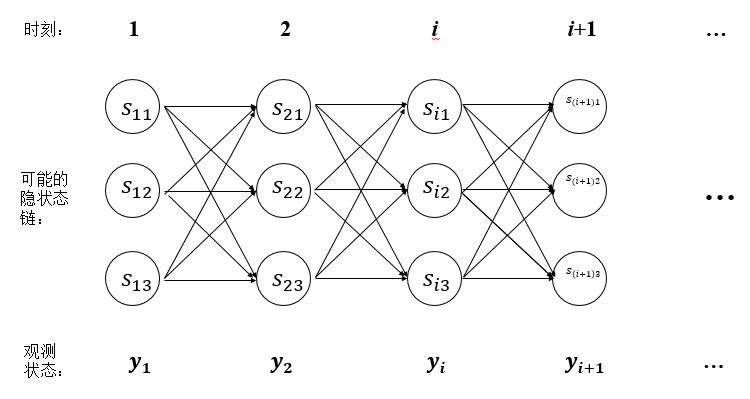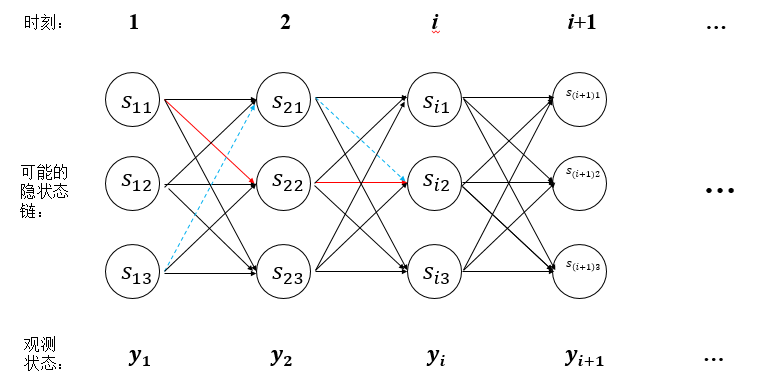$j$$i$的转移概率，再乘上$i$发射$y_{t+1}$的概率。

$t=1$时，$y_1=1$，有$\delta_1(A)=0.6*1/6=0.1$$\delta_1(B)=0.4*0.1=0.04$

$t=2$时，$y_2=3$，有：

1. 隐状态为A：a）A->A有$\delta_2(A)=(1/6)*0.1*0.8=1.33*10^{-2}$；b）B->A有$\delta_2(A)=(1/6)*0.04*0.1=6.6*10^{-4}$。所以A->A，$\psi_2(A)=A$
2. 隐状态为B：a）A->B有$\delta_2(B)=0.1*0.1*0.2=2*10^{-3}$；b）B->B有$\delta_2(B)=0.1*0.04*0.9=3.6*10^{-3}$。所以B->B，$\psi_2(B)=B$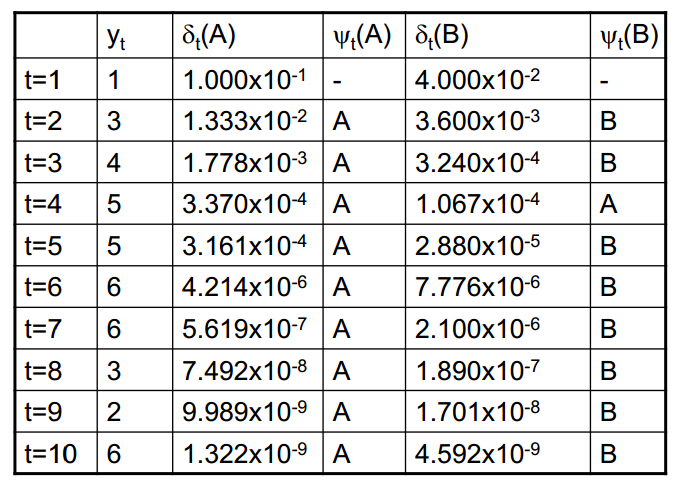$t=10$时最大概率为$\delta_{10}(B)$，经过回溯得到最佳隐状态为：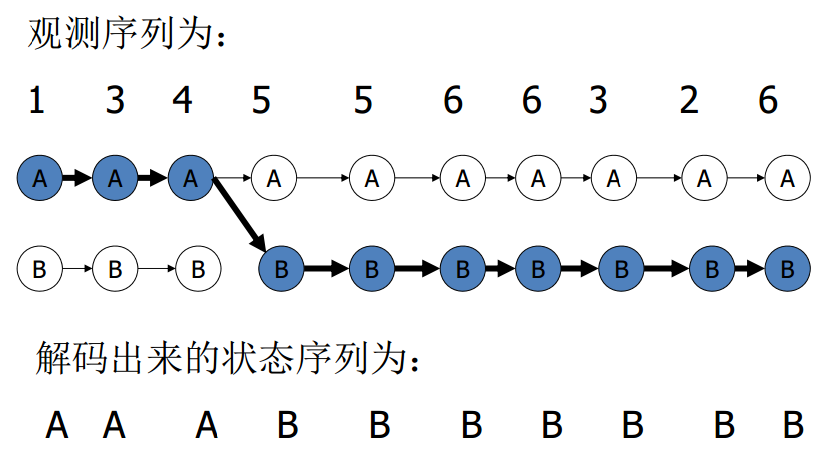Viterbi算法还可用于解决语音识别或者拼音输入法。我们知道中文的一个拼音可以对应多个汉字，连续的一段拼音就能组成成千上万种可能的句子，哪一个句子才是最佳候选呢？我们可以把每个拼音当成观测状态，同音的汉字当成可能的隐状态。通过背景语料库统计得到每个汉字出现在词首的概率、汉字之间的转移概率和汉字与拼音之间的发射概率，这样我们就能得到模型参数，然后利用Viterbi算法求解出一个最佳的隐状态序列，这样就能完成一个简易的拼音输入法。

HMM在实际中主要有3个方面的应用，分别是：

1. 从一段观测序列$\mathbf{Y}$及已知模型$\mathbf{\lambda=(\mu,A,B)}$出发，估计出隐状态$\mathbf{X}$的最佳值，称为解码问题，这是状态估计问题。这篇博客讨论的就是这个问题。
2. 从一段观测序列$\mathbf{Y}$出发，估计模型参数组$\mathbf{\lambda=(\mu,A,B)}$，称为学习问题，就是参数估计问题。
3. 对于一个特定的观测链$\mathbf{Y}$，已知它可能是由已经学习好的若干模型之一所得的观测，要决定此观测究竟是得自其中哪一个模型，这称为识别问题，就是分类问题。

This site uses Akismet to reduce spam. Learn how your comment data is processed.